# SSAT Upper Level Math : Acute / Obtuse Triangles

## Example Questions

← Previous 1 3 4 5 6 7 8 9

### Example Question #1 : How To Find If Acute / Obtuse Triangles Are Congruent

If the vertex angle of an isosceles triangle is 30 degrees, what is the value of the angle that is exterior to one of the base angles?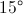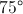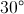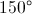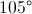Explanation:

First, you subtract one angle so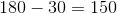. Because there are 2 base angles you divide that value by 2 to get 75 Degrees. The exterior angle and the base angle are supplementary angles so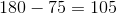.

### Example Question #1 : Acute / Obtuse Triangles

The side lengths of a triangle are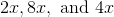. Find the perimeter of the triangle.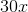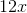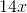Explanation:

To find the perimeter of a triangle, add up its side lengths.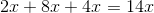### Example Question #3 : Acute / Obtuse Triangles

The side lengths of a triangle, in inches, are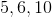. Find the perimeter of this triangle.Explanation:

Find the perimeter of a triangle by adding up all its sides.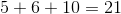### Example Question #4 : Acute / Obtuse Triangles

A triangle has side lengths, and. What is the perimeter of this triangle?Explanation:

To find the perimeter of a triangle, add up all the side lengths.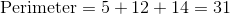### Example Question #5 : Acute / Obtuse Triangles

In terms of, find the perimeter of a triangle with side lengths of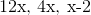.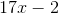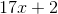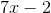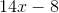Explanation:

To find the perimeter of a triangle, add up all of its sides.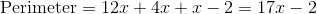### Example Question #1 : Acute / Obtuse Triangles

In terms of, find the perimeter of a triangle with side lengths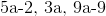.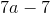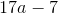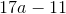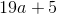Explanation:

To find the perimeter of a triangle, add up all of its sides.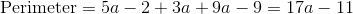### Example Question #7 : Acute / Obtuse Triangles

In terms of, find the perimeter of a triangle with side lengths of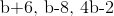.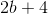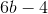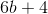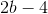Explanation:

To find the perimeter of a triangle, add up all of its sides.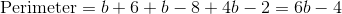### Example Question #8 : Acute / Obtuse Triangles

In terms of, find the perimeter of a triangle with side lengths of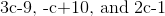.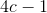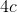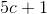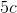Explanation:

To find the perimeter of a triangle, add up all of its sides.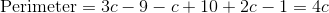### Example Question #9 : Acute / Obtuse Triangles

Find the perimeter of a triangle with side lengths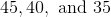.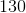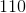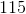Explanation:

To find the perimeter of a triangle, add up all of its sides.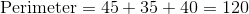### Example Question #10 : Acute / Obtuse Triangles

Bill has a triangular garden that he needs to fence. The garden has side lengths of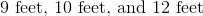. In feet, how much fencing will Bill need?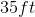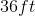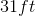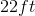Explanation:

To find how much fencing Bill needs, you will need to find the perimeter of the triangle. The perimeter of a triangle is found by adding up all the sides together.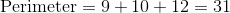← Previous 1 3 4 5 6 7 8 9

### All SSAT Upper Level Math Resources